Home » Quizzes » What’s the value of this note?

# What’s the value of this note?

Select the right value for each note.

1.
What's the value of this note?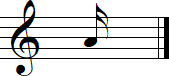2.
What's the value of this note?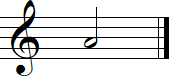3.
What's the value of this note?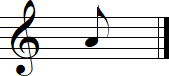4.
What's the value of this note?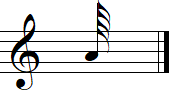5.
What's the value of this note?6.
What's the value of this note?7.
What's the value of this note?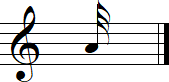8.
What's the value of this note?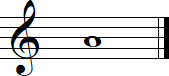9.
What's the value of this note?10.
What's the value of this note?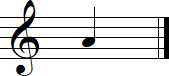11.
What's the value of this note?12.
What's the value of this note?13.
What's the value of this note?Share!

## 2 thoughts on “What’s the value of this note?”

1. I enjoyed this quiz. I’m getting back into reading music and need the practice.
Thanks,
Ed P.

1. You’re welcome, Ed!